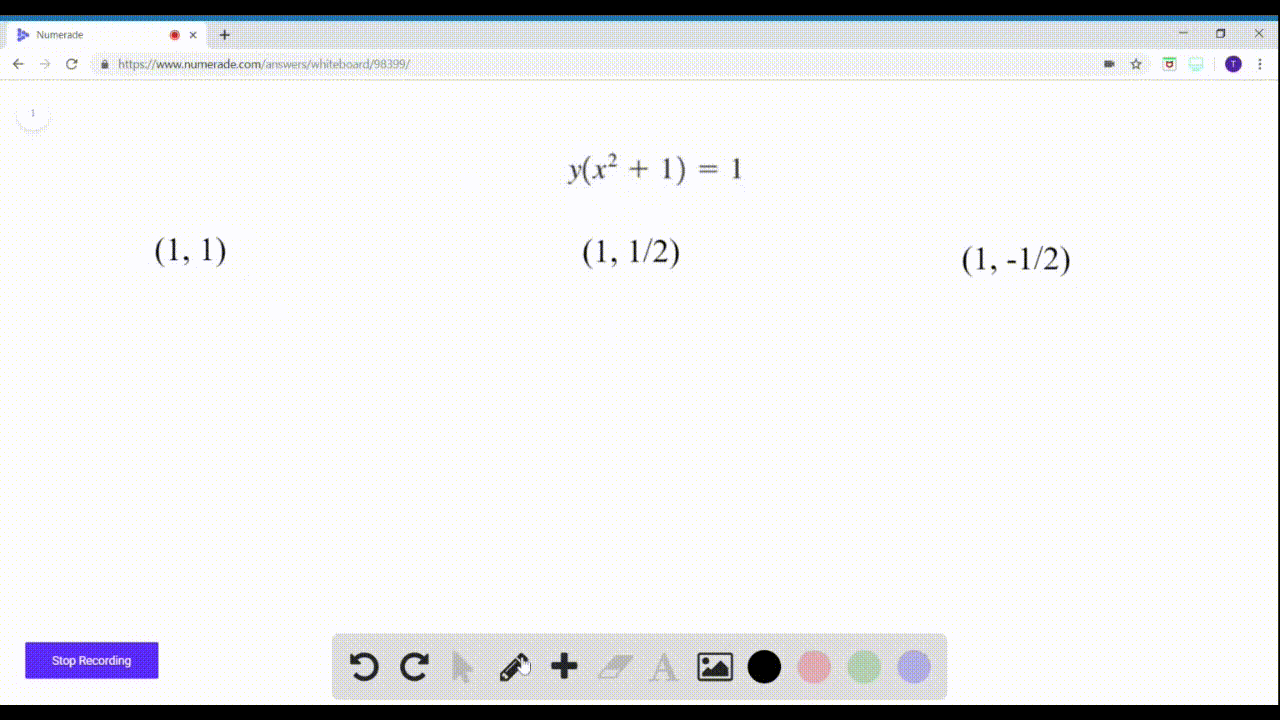🎉 The Study-to-Win Winning Ticket number has been announced! Go to your Tickets dashboard to see if you won! 🎉View Winning Ticket### $9-14=$ Points on a Graph? Determine whether the …

01:59Problem 11

# $9-14=$ Points on a Graph? Determine whether the given pointsare on the graph of the equation.$$x-2 y-1=0 ; \quad(0,0),(1,0),(-1,-1)$$

## Discussion

You must be signed in to discuss.

## Video Transcript

for this item were asked to determine if these three points lie on the graph of the equations shown in turn. We will substitute the coordinates given into the equation. Do the math. Here we find that negative one is not equal. 000 is not in the graph. You continue will do one minus two. I'm zero minus one equal zero one minus one equals zero zero equals zero. Yes, the 0.10 is on the graph of the given equation. And finally, let's substitute in native one. Whoops. So just that negative one minus two times negative. One minus one. Does it equal zero native one plus two. Honest one equals zero gathered the negatives. Negatives too. Plus two equals zero zero equals zero. And again, we have a true statement. So we know that negative one negative one is on the graph of the given equation.